Next: Floating Point arithmetic with Up: Approaches to Real Arithmetic Previous: Approaches to Real Arithmetic

Floating Point Arithmetic

Conventionally, floating point arithmetic has been used to perform real arithmetic with computers, and floating point operations have been highly studied and optimised. The standard most commonly used is IEEE-754, which includes 32-bit single' precision and 64-bit double' precision representations.

Floating point represents a number as fixed length binary mantissa and an exponent of fixed size. This representation only allows numbers in a finite sub-interval of the whole real line to be represented, and only a finite number of the elements within that interval to be represented exactly.

The major problem with floating point arithmetic is that it is inherently inaccurate. There are two reasons for this. Firstly because only a finite subset of real numbers may be represented exactly, it is necessary to approximate all other numbers by the nearest representable one. Secondly, every time a floating point operation is performed, rounding occurs, and the rounding error introduced can have very serious effect on the accuracy of the result.

If several floating point operations are performed, carrying the result from one to the next, the rounding error is propagated. In some cases, this can lead to the computed result being entirely `noise' and bearing no apparent relation to the actual one.

Other problems with floating point arithmetic include the fact that the floating point arithmetic operations and transcendental functions do not correspond directly to the mathematical ones, in addition to which certain mathematical laws such as associativity do not hold.

One dramatic demonstration of the potential inadequacy of floating point arithmetic is to observe the effects of computing successive iterations of the so called logistic map using floating point arithmetic, and then observing how the computed result differs from the correct one.

The logistic map is defined as f(x) = Ax(1-x) where A is a real constant. The iterated logistic map, which is also known as the Verhulst Model after it was published in 1845 by the Belgian mathematician Pierre Verhulst as a model of population growth, exhibits chaotic behaviour when certain values for the constant A are used.

We let A=4, and x to be the following simple (arbitrary but machine representable) number. The subscripts here denote the base of the representation.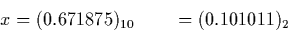The logistic map function is now iterated repeatedly using single and double precision arithmetic. The correct result (computed using the exact real arithmetic functions implemented as part of this work) is shown in the right hand column. All correct digits are underlined.

 It. Single Precision Double Precision Correct Result 15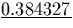1015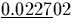20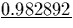25 0.65283730 0.934927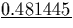40 0.05769650 0.042174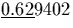60 0.934518 0.757154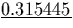One can clearly see how very rapidly the results computed using floating point arithmetic become incorrect. Increasing the size of the mantissa clearly improves matters, but the same problems occur, and simply increasing the size of the mantissa does not necessarily guarantee that any given computation is correct. Valérie Ménissier-Morain  explores these problems further.Next: Floating Point arithmetic with Up: Approaches to Real Arithmetic Previous: Approaches to Real Arithmetic
Martin Escardo
5/11/2000